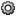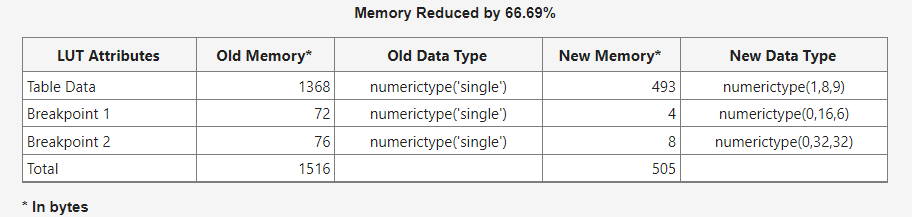Main Content

## Optimize Lookup Tables for Memory-Efficiency

The Lookup Table Optimizer optimizes the spacing of breakpoints and the data types of lookup table data to reduce the memory used by a lookup table. Using the Lookup Table Optimizer and its command-line equivalent, you can:

• Optimize an existing Lookup Table block.

• Generate a lookup table from a Simulink® block, including a Math Function block or a subsystem.

• Generate a lookup table from a function or function handle.

### Optimize an Existing Lookup Table Using the Lookup Table Optimizer

To optimize an existing lookup table, open the model containing the Lookup Table block.

```load_system('sldemo_fuelsys'); open_system('sldemo_fuelsys/fuel_rate_control/airflow_calc');```
This example shows how to optimize the `Pumping Constant` Lookup Table block.

1. To open the Lookup Table Optimizer, select the `Pumping Constant` Lookup Table block. The context-sensitive Lookup Table tab appears in the Simulink toolstrip. In the Lookup Table tab, select Lookup Table Optimizer.

2. Select the type of block you want to optimize. To optimize a Simulink block or subsystem, including an existing Lookup Table block or a Math Function block, select Simulink block or subsystem. To generate a lookup table approximation for a function handle, select MATLAB Function Handle.

In this example, select Simulink block or subsystem to optimize the `Pumping Constant` lookup table. Click .

3. Under Block Information, enter the path to the ```Pumping Constant``` Lookup Table block. Select the block in the model, then click in the Lookup Table Optimizer to fill in the block path automatically.

4. Click to update the model diagram and allow the Lookup Table Optimizer to automatically gather information needed for the optimization process including current output data type, and input number, data type, and value range. You can manually edit all of these fields to specify ranges and data types other than those currently specified on the block.

• Specify the Desired Output Data Type of the generated lookup table as a `numerictype` or `Simulink.NumericType` object.

• Specify the data type of each input to the block as a `numerictype` or `Simulink.NumericType` object.

• Specify the minimum and maximum values of each input of the generated lookup table as scalars in the table.

For this example, use the current values specified on the model. Click .

5. Specify constraints to use in the optimization. Set the Output Error Tolerance that is acceptable for your design.

• Absolute tolerance is defined as the absolute value of the difference between the original output value and the output value of the optimized lookup table.

• Relative tolerance measures the error relative to the value at that point, specified as a non-negative.

6. Specify the allowed word lengths as a vector based on types that are efficient for your intended hardware target. For example, if you want to allow the optimizer to consider only 8-, 16-, and 32-bit types, specify `[8 16 32]` in the Allowed Word Lengths (Vector) field.

7. To specify additional properties for the optimized lookup table, click . For more information on each of the properties, see `FunctionApproximation.Options`. In this example, use the default values for these properties.

8. Specify options for the optimization, such as the maximum time or maximum memory usage for the generated lookup table by clicking thebutton.

9. After you set the constraints, click .

Optionally, you can choose to stop the optimization solver before the optimization solver is complete by clicking Stop. The optimizer will choose the best solution found at the time the Stop button is selected and display it in the app.

When the optimization is complete, the optimizer reports the memory of the optimized lookup table. You can edit the constraints and run the optimization again to achieve further memory reduction.

Using the default settings, the Lookup Table Optimizer reduces the memory used by the `Pumping Constant` Lookup Table block from 1516 bytes to 505 bytes (66.69%).Click .

10. Click to view a plot of the original block output compared to the output of the new optimized lookup table.Click to generate a new lookup table using the optimized settings found by the app, and replace the original block.

The new block is a masked variant subsystem in which the active variant is the optimized lookup table block. The inactive variant is the original block.### Edit the Optimization Settings and Generate a New Approximate

You can iteratively change the approximation block by editing the settings used during the optimization to generate a new lookup table.

1. Double-click the Pumping Constant block. To edit the optimization settings, in the Block Parameters dialog, click .

2. In the Lookup Table Optimizer, click to proceed to the Create page of the app. In this example, edit the absolute and relative tolerances to a slightly larger value so that you can further reduce the size of the lookup table.

• Set the Absolute tolerance to `0.01`, or 1%.

• Set the Relative tolerance to `0.01`, or 1%.

3. Click to optimize the lookup table with the new options.

Using these tolerance values, the new lookup table uses only 304 bytes of memory.

4. Click . On the Results page, click the button to replace the first iteration of the approximation block with this newest iteration.

5. In the model, double-click the Pumping Constant block to open the Block Parameters. The Block Parameters displays the settings used for the approximation

To make the original block or subsystem the active variant, next to Select desired function version, select `Original`.

To delete the lookup table approximation from the model, in the Block Parameters, click Revert to original.

## SupportGet trial now